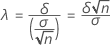# Methods and formulas for Power and Sample Size for Paired t

Select the method or formula of your choice.

## Calculating power

These calculations rely on a t-distribution with a noncentrality parameter λ.

### Noncentrality parameter### One-sided power (H1: μd < 0)

Power = t(–tα; v, λ)

### One-sided power (H1: μd > 0)

Power = 1 – t(tα; v, λ)

### Two-sided power (H1: μd ≠ 0

Power = 1 – t(tα/2; v, λ) + t(–tα/2; v, λ)

### Notation

TermDescription
μd population mean of the differences between paired observations
TermDescription
λ noncentrality parameter for t
δ difference
σ standard deviation of paired differences
n sample size
t(x; v, λ) CDF of the t distribution with v degrees of freedom and noncentrality parameter λ evaluated at x
tα one-sided critical value (upper α point of the t distribution with v degrees of freedom)
t α/2 two-sided critical value (upper α / 2 point of the t distribution with v degrees of freedom)
v degrees of freedom for error = n– 1

## Calculating sample size and difference

If you provide values for power and sample size, Minitab calculates the value of the difference. If you provide values for power and difference, Minitab calculates the value of the sample size.

For these two cases, Minitab uses an iterative algorithm with the power equation. At each iteration, Minitab evaluates the power for a trial sample size or trial difference value, and stops when it reaches the values you request.

### Target power and actual power

When Minitab calculates sample size, it may find that no integer value of sample size yields your target power. In such cases, Minitab displays the target value for power alongside the actual power, which is a value corresponding to an integer sample size, and which is nearest to, yet greater than, the target value.

By using this site you agree to the use of cookies for analytics and personalized content.  Read our policy